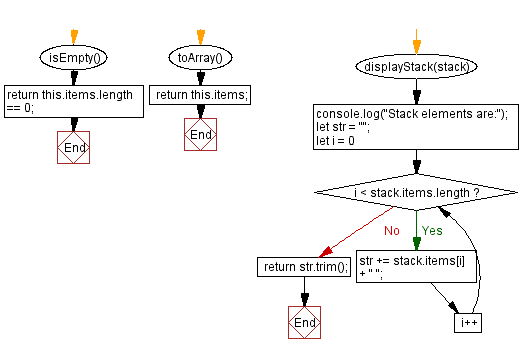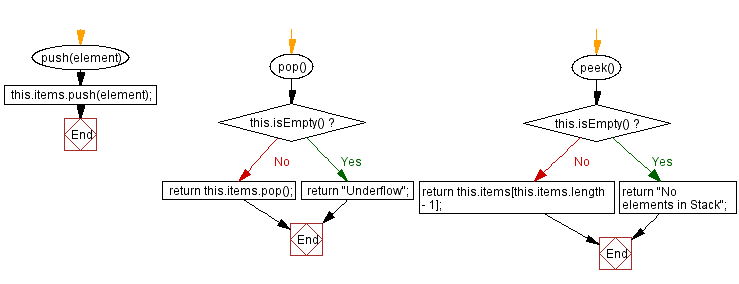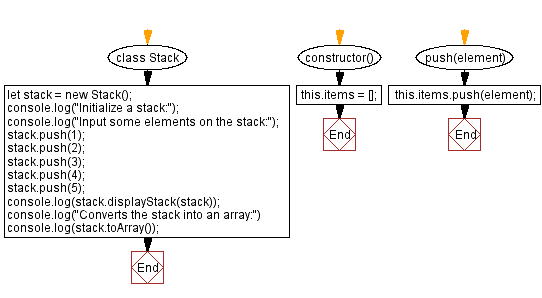# JavaScript Exercises: Convert a stack into an array

## JavaScript Stack: Exercise-22 with Solution

Write a JavaScript program to implement a stack that supports toArray() operation, which converts the stack into an array.

Sample Solution:

JavaScript Code:

``````class Stack {
constructor() {
this.items = [];
}

push(element) {
this.items.push(element);
}

pop() {
if (this.isEmpty()) {
return "Underflow";
}
return this.items.pop();
}

peek() {
if (this.isEmpty()) {
return "No elements in Stack";
}
return this.items[this.items.length - 1];
}

isEmpty() {
return this.items.length == 0;
}

toArray() {
return this.items;
}

displayStack(stack) {
console.log("Stack elements are:");
let str = "";
for (let i = 0; i < stack.items.length; i++)
str += stack.items[i] + " ";
return str.trim();
}

}

let stack = new Stack();
console.log("Initialize a stack:");
console.log("Input some elements on the stack:");
stack.push(1);
stack.push(2);
stack.push(3);
stack.push(4);
stack.push(5);
console.log(stack.displayStack(stack));
console.log("Converts the stack into an array:")
console.log(stack.toArray());
```
```

Sample Output:

```Initialize a stack:
Input some elements on the stack:
Stack elements are:
1 2 3 4 5
Converts the stack into an array:
[1,2,3,4,5]
```

Flowchart:Live Demo:

See the Pen javascript-stack-exercise-22 by w3resource (@w3resource) on CodePen.

Improve this sample solution and post your code through Disqus

Stack Previous: Implement a stack using a linked list.
Stack Exercises Next: Create stacks from arrays.

What is the difficulty level of this exercise?

Test your Programming skills with w3resource's quiz.

﻿